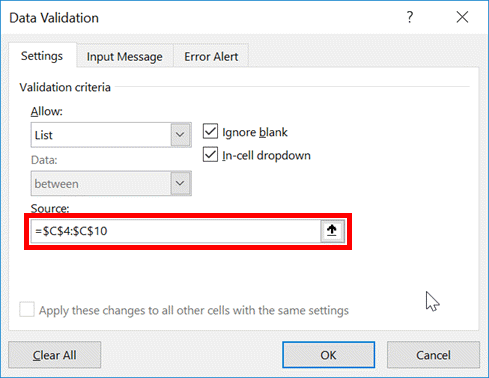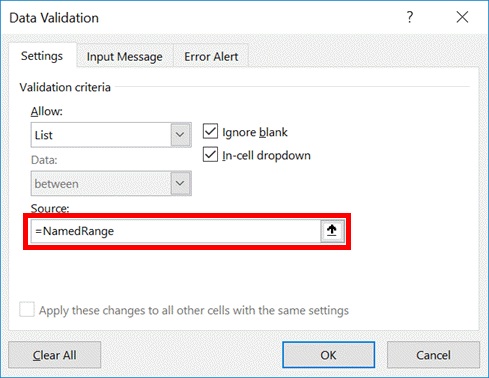Get our FREE VBA eBook of the 30 most useful Excel VBA macros.

Automate Excel so that you can save time and stop doing the jobs a trained monkey could do.

# Loop through each item in a Data Validation list with VBAI had an interesting question from a reader recently.  He wanted to save a PDF for each item in a data validation drop-down list.  In the past, when I have tackled similar tasks, I have always used a named range with all the possible values, then looped through the cells in the named range.

My interest was piqued, by the inclusion of the data validation drop-down list.  Below you will find my approach to looping through the list of options.

## Types of data validation drop-down

Not all data validation lists are the same.  They can be based on a:

• Standard cell range
• Named range
• Comma-separated list

The screen shows below illustrate how these three methods are set up.

Cell rangeNamed rangeComma-separated listThe cell range and named range methods can be treated the same way from a VBA perspective, as they are both ranges. A comma-separated list is slightly different and requires an alternative approach.  The code below covers both of these options.

## The VBA Code

The code below will loop through each item in a data validation list, no matter which type.

```Sub LoopThroughDataValidationList()

Dim rng As Range
Dim dataValidationArray As Variant
Dim i As Integer
Dim rows As Integer

'Set the cell which contains the Data Validation list
Set rng = Sheets("Sheet1").Range("B2")

'If Data Validation list is not a range, ignore errors
On Error Resume Next

'Create an array from the Data Validation formula, without creating
'a multi-dimensional array from the range
rows = Range(Replace(rng.Validation.Formula1, "=", "")).rows.Count
ReDim dataValidationArray(1 To rows)

For i = 1 To rows
dataValidationArray(i) = _
Range(Replace(rng.Validation.Formula1, "=", "")).Cells(i, 1)
Next i

'If not a range, then try splitting a string
If Err.Number <> 0 Then
Err.Clear
dataValidationArray = Split(rng.Validation.Formula1, ",")
End If

'Some other error has occured so exit sub
If Err.Number <> 0 Then Exit Sub

'Reinstate error checking
On Error GoTo 0

'Loop through all the values in the Data Validation Array
For i = LBound(dataValidationArray) To UBound(dataValidationArray)

'Change the value in the data validation cell
rng.Value = dataValidationArray(i)

'Force the sheet to recalculate
Application.Calculate

''''''''''''''''''''''''''''''''''''''''''
'Insert the code here'''''''''''''''''''''
''''''''''''''''''''''''''''''''''''''''''

Next i

End Sub```

If it is the PDF aspect which you are specifically interested in, then check out my post about saving Excel files as PDF with VBA.

## 6 thoughts on “Loop through each item in a Data Validation list with VBA”

1.Hello,

How can I loop through data in an excel spreadsheet and grab each cells data value one at a time? Then, I want to check to see if it is in a temperature range and then output to a new worksheet. My worksheet has 21 columns and 400 rows.

Thanks!

2.Jay says:

Hi Excel off the Grid, I would be able to save so much time if I combined the above script with a part that includes saving each of the data validation options as a separate PDF in the same folder as the Excel file (naming it the name of the data validation option). I checked out your other feature on Saving Excel Files as a PDF, but I am not proficient enough to combine the two into a usable script. Can you please assist? Thank you so much

3.cc says:

I have 5 worksheets set up to one data validation list in cell A6. I need to print each one of these as a PDF or preferably an Excel workbook. Could someone assist me with this code?

4.Suci says:

hi thankyou for your code, but what do you mean with Formula1? should i replace the formula1? i tried with only change my sheet dan range name but i only got 0.pdf. please help thankyou

‘Create an array from the Data Validation formula, without creating
‘a multi-dimensional array from the range
rows = Range(Replace(rng.Validation.Formula1, “=”, “”)).rows.Count
ReDim dataValidationArray(1 To rows)

•Excel Off The Grid says:

Formula1 is the property from the Document Object Model. It should work by changing the sheet and range name.